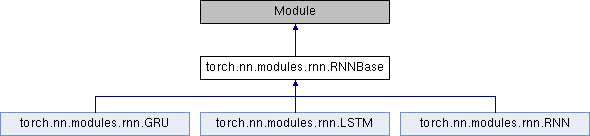Caffe2 - Python API A deep learning, cross platform ML framework
torch.nn.modules.rnn.RNNBase Class Reference
Inheritance diagram for torch.nn.modules.rnn.RNNBase:## Public Member Functions

def __init__ (self, mode, input_size, hidden_size, num_layers=1, bias=True, batch_first=False, dropout=0., bidirectional=False)

def flatten_parameters (self)

def reset_parameters (self)

def get_flat_weights (self)

def check_input (self, input, batch_sizes)

def get_expected_hidden_size (self, input, batch_sizes)

def check_hidden_size (self, hx, expected_hidden_size, msg='Expected hidden size{}, got)

def check_forward_args (self, input, hidden, batch_sizes)

def permute_hidden (self, hx, permutation)

def forward (self, input, hx=None)

def extra_repr (self)

def __setstate__ (self, d)

def all_weights (self)

mode

input_size

hidden_size

num_layers

bias

batch_first

dropout

bidirectional

## Detailed Description

Definition at line 28 of file rnn.py.

## Member Function Documentation

 def torch.nn.modules.rnn.RNNBase.flatten_parameters ( self )
```Resets parameter data pointer so that they can use faster code paths.

Right now, this works only if the module is on the GPU and cuDNN is enabled.
Otherwise, it's a no-op.
```

Definition at line 94 of file rnn.py.

The documentation for this class was generated from the following file: